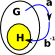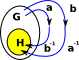# Maths - Subgroups and Factoring Groups

### Types of 'Division'

On these pages we will discuss these types of 'division' of groups to produce a smaller group:

• Subgroup
• Quotient Group (or Factor Group)

Quotient groups and subgroups are both ways of forming a smaller group from a larger one. In category theory terms they are duals of each other.

### Extension Problem

Unlike conventional multiplication and divisions, where the result of a multiplication can be divided back to give the original operands, these group operations can not always be reversed in this way (although in many cases they can).

An example of where it is not possible is: C4, a cyclic group of order 4, such as complex numbers which can be generated by 'i' which generates the cycle 1 i -1 -i. We can divide this by C2, for instance '-1' which generates the subgroup 1 -1 . However we cannot reverse this and generate C4 from C2C2 and the semidirect and bicrossed products are identical to the direct product because reversing the direction of arrows in the Cayley graph does not change it.

### Subgroup

Lets assume that we have a group G and we have a subgroup of G which we call N. We want to find the other subgroup G/N. For G/N to be a group then N must be a normal subgroup. Sometimes, but not necessarily, a group G can be reconstructed from G/N and N, as a direct, semidirect or bicrossed product.

## Quotient Group

A quotient group (or factor group) is a group obtained by identifying together elements of a larger group using an equivalence relation.

For example, the cyclic group of addition modulo 5 can be obtained from the integers by identifying elements that differ by a multiple of 5.In a quotient of a group, the equivalence class of the identity element is always a normal subgroup of the original group, and the other equivalence classes are the cosets of this normal subgroup. The resulting quotient is written G / N, where G is the original group and N is the normal subgroup.### Test that N is a normal subgroup

To test that N is a normal subgroup then:

g N g -1 must be a member of N for every g in G

### Calculation of G/N

we calculate the set:

gN | gG

This will be the group G/N under the operation

(g1 N) (g2 N) = g1 g2 N

### Proof

let H = G/N

to prove that H is a group we must prove that:

 H is closed under multiplication (h1 N) (h2 N) = h1 h2 N h-1 exists (hN) (h-1 N) = 1

We will represent multiplication by concatenating into a single word:

(h1 N) (h2 N) = h1 N h2 N

but since h is an element of G then: h2N = N h2

=h1h2 N N

Since N is a group it is closed under multiplication. Taking and example element n from N then the produce N N will be n' n

## Group Equivalences

The way that left or right cosets of a subgroup partition a group is an equivalence relation. See page here.

let:

• H be a subgroup of group G.
• ~ be an equivalence relation on G
• a ~ b ↔ (ab−1H).
Group Equivalence Relationx~y
transitivea b-1b c-1=a c -1

since b-1b=1

x~y, y~zx~z

(composition law)

reflexiveif a maps to H then composing with 1 wont change that.

x~x
symmetricThe inverse of a b-1 is b a-1

If H contains a b-1 then it must contain its inverse.

x~yy~x

(symmetry law)

Where I can, I have put links to Amazon for books that are relevant to the subject, click on the appropriate country flag to get more details of the book or to buy it from them.The Princeton Companion to Mathematics - This is a big book that attempts to give a wide overview of the whole of mathematics, inevitably there are many things missing, but it gives a good insight into the history, concepts, branches, theorems and wider perspective of mathematics. It is well written and, if you are interested in maths, this is the type of book where you can open a page at random and find something interesting to read. To some extent it can be used as a reference book, although it doesn't have tables of formula for trig functions and so on, but where it is most useful is when you want to read about various topics to find out which topics are interesting and relevant to you.